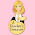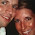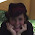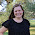## Monday, May 14, 2012

### MNM: Area of Trapezoids... WHY??

WHY?!?!

The most intriguing question that I ask and try to have an answer for.  So I was about to teach how to find area of a trapezoid, and I thought I could just teach them the formula and how to use it, but then the question hit me... Why??  Why is the formula like this???

My students had a formula chart we refer to as the "cheat sheet" (they get to use this on their STAAR test) and I knew I would not be a good teacher if I didn't explain why the formula was the way it was.

My students already had figured out the formula to finding the area of a triangle: bh/2 because a triangle is 1/2 of the area of a rectangle or square.

They figured that out all on their own... with a little jogging by me.  But I had NO IDEA why the formula to a trapezoid was (Base 1 + Base 2)h/2.  Why was the formula this?? And if I couldn't figure it out, how on earth could I teach it???  AHHHH....

So I sat at the kitchen table with my husband and drew, thought, scribbled, reassessed, guess and checked and learned all over again.  The divide by 2 made me think... somehow a triangle is involved here....Then it hit me.... duh... 2 triangles make up a trapezoid.... but they have different bases.  If I find the area of each one and add them then I can find the area.  And it worked.

So I used my jogging questions to help my students figure out the formula to a trapezoid. We started with a trapezoid cut out that I provided to all my students.  I told them that triangles and trapezoids were related.  Using one line, can you figure out how and draw it?  Gotta admit... it stumped some them... they really wanted to draw a square and a triangle(just like I did the night before), but soon enough the light started turning on and they drew a line cutting the trapezoid into 2 triangles.
I told them they already knew how to find the area of triangles, so what could we do to find the area of the trapezoid?  ( Find the area of each triangle and add them)  And so they did.  You can see the work in each triangle and the adding of the 2 triangle areas in the bottom right hand corner of the journal page.

Once they understood this concept, I told them there was an easier way then finding the area of both triangles and adding.  I asked what did the 2 triangles have in common? (the height) What was different in both triangles? (the bases) Well both the bases are being multiplied by the height. right... couldn't we just add the bases and multiply them by the height since they are going to anyway?  Lets test it and see what happens....
Sure enough... we got the same answer.  So why can we do this?  (both of the bases are being multiplied by the same number)  Then I asked what did they notice about the 2 bases? what kind of lines were they? (parallel)  So how can you always find the bases in a trapezoid?  (look for the parallel lines)

With much practice, they can do the formula in no time, but even more importantly, when I ask them why we add the bases, or divide by 2, they can tell me exactly why.  Warm feeling filling the heart now...

And on a side note.... didn't I learn all this in 10th grade Geometry???? When did 10th grade become 6th grade math!??!?!

Gotta get my warm heart feeling again after that blood pressure spike....

Happy Teaching the answer to WHY???

#### 6 comments:

1.Yey Leslie!!!! You're back to blogging :) We've missed ya!

2.I've missed yall too!!! I am trying to get back in the habbit:) Looking forward to daily blogging this summer:)

3.Hello!

Thanks for giving your inspiring ideas :)

Another awesome way to teach the area of a trapezoid is to make a perfect copy of the trapeziod, turn it upside down and attach it on to the first one. Because two of the sides are parallel the two shapes will always give you a parallelogram. And if you teach the area of a parallelogram first (base times perpendicular height) then it is easy to conclude that the area of the trapezoid is half of the base (of the whole parallelogram, which is one "top" and one "bottom") multiplied by the perpendicular height!

Hope that makes sense.

4.A trapezium has no parallel sides and any quadrilateral drawn randomly would probably be a trapezium and if the quadrilateral had one pair of parallel sides then it is a trapezoid and if both pairs of sides are parallel its a parallelogram.
Formula: Average width × Altitude
how to find the domain of a function

5.Thank you for the help!! I was struggling in how to connect this well for my students to brainstorm then lead into the connection to a parallelogram.

6.Yes, now 6th grade math has become geometry and Algebra 1. It makes you wonder what is being taught in those classes now!